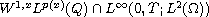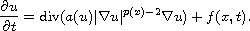Electron. J. Diff. Equ., Vol. 2016 (2016), No. 209, pp. 1-15.

### Weak solutions for parabolic equations with p(x)-growth Ning Pan, Binlin Zhang, Jun Cao

Abstract:
In this article we study nonlinear parabolic equations with p(x)-growth in the space. By using the method of parabolic regularization, we prove the existence and uniqueness of weak solutions for the equationAlso, we study the localization property of weak solutions for the above equation.

Submitted April 2, 2016. Published August 2, 2016.
Math Subject Classifications: 35K15, 35K20, 35K55.
Key Words: Parabolic equation; p(x)-growth condition.

Show me the PDF file (282 KB), TEX file for this article.Ning Pan Department of Mathematics Northeast Forestry University Harbin 150040, China email: hljpning@163.com Binlin Zhang Department of Mathematics Heilongjiang Institute of Technology Harbin 150050, China email: zhangbinlin2012@163.com Jun Cao College of Mechanical and Electrical Engineering Northeast Forestry University Harbin 150040, China email: zdhcaojun@163.com